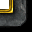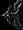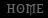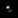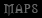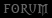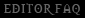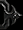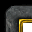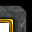# vJass - I: Uncomplicating the Complicated

Tutorial By Romek

vJass - I: Uncomplicating the Complicated
By Romek - The First in the Series

Introduction:This tutorial is best viewed on Thehelper forums. If you are viewing this tutorial from the World-Editor-Tutorials website, click here.

This tutorial is the first in a series of vJass tutorials which I will be writing. The tutorials are ultimately aimed at wannabe spell and system makers, though anyone can use them. This first tutorial will go over the very basics of vJass, and will not be covering resource making.
I know, another Jass tutorial (:(). Though I have to start my series from something, don't I? And maybe this one will cover things that other's didn't.

This tutorial should cover all the basics of vJass, including functions, variables, arguments, scopes, etc. We'll also cover removing BJs*, which is an essential skill for any coder. I suggest trying these out as you go along, making code and seeing if it compiles, and possibly testing it. :)

I also suggest taking breaks throughout this tutorial, as it can be a lot for one go. If you have any questions or suggestions, be sure to post them here!

* - BJs are basically useless functions. Will be explained later in more detail.

Starting Jass:Create a trigger object, go to Edit -> Convert to Custom Text, and delete everything that's there. This is the place where you'll be vJassing.

An important rule I think I should mention here, before anything else, is that Jass is CaSe SeNsItIvE!. So make sure you keep that in mind!

Triggers in JassWhile using GUI, you have learned that a trigger is something that contains events, conditions and actions, and you can browse triggers by looking at the little tree view on the left side of the editor. This is actually a false idea of what triggers actually are. Triggers, are actually just another variable type, just like effects or unit groups. The things you see on the left side will be referred to as 'Trigger Objects' throughout this tutorial, to not get the two ideas mixed up.

Instead of Events, Conditions and Actions, Jass actually uses functions and initializers.
Initializers are functions that are called when the map is loading. This is where triggers are created, and events, conditions and actions are added to them. It's very possible to have a trigger object without a single trigger, as they are most certainly not a necessity.

Functions and Comments:Jass is coded entirely using functions. Everything you see and do is made through functions. To declare a function, use the following syntax:
```function NAME takes nothing returns nothing
// Things inside the function
endfunction```

NAME is obviously the name of the function. Functions can be called anything you want them to, as long as it doesn't start with a number (or underscore), and contains only letters, numbers or underscores (_).
Here are some examples of Valid function names:
• Init (Only letters)• Hello_World (Underscores are allowed)• B4N4N4PI3 (Numbers are allowed if they're not the first character)
Whilst the following are Invalid:
• 3Hello (Numbers first are disallowed)• Find% (Uses non alphanumeric characters)

If functions aren't initializers, they must be called using the following syntax:
`call NAME()`

NAME is the name of the function. You cannot call a function that's below the place you're calling from in Jass.
```function A takes nothing returns nothing
call B() // Will give an error. B is below A.
endfunction

function B takes nothing returns nothing
call A() // Will make \'function A\' run.
endfunction```

Notice the comment in the middle of the function. Everything written after a double slash (//) will be ignored by the parser, so you can type anything you want there to make your code look cleaner, or to leave notes for yourself. I strongly suggest you use comments as much as possible, as it certainly helps people understand your code. Also, if you've left some code for a month or so, and come back to it. You'll have a difficult time grasping what everything does if you don't comment.
A good rule to remember while commenting things is that you should always write why you are doing something, and not what you are doing.
``` - Bad: -
call RemoveLocation(loc) // removing location

- Good: -
call RemoveLocation(loc) // preventing leaks and lag```

Variables:You should already know what a Variable is from your GUI experience. However, there are two types of variable in Jass. The type of variable you used in GUI is called a Global. This is because it can be accessed from anywhere in the script (or scope), and can be overwritten from anywhere. To declare a global in vJass, use the following syntax:
```globals
TYPE NAME         // 1
TYPE NAME = VALUE // 2
integer Five      // 3
integer Four = 4  // 4
endglobals```

The globals commented with 1 and 2 are the basic syntax for globals, and will not compile (save) as they are now. 3 and 4 are both examples, which will compile without errors. TYPE can be any variable type, for example: unit, integer and effect.

Some notable types are integer, real, boolean and string. An integer is a whole number; a real is a number with a decimal point; a boolean is either true or false; and a string is a set of characters, or a message.
What's also notable is that integers can be in hexadecimal and octal. Here are some example usages of these common types:
```local boolean b = true
local real r = 0.47249
local integer i1 = 90429 // Decimal
local integer i2 = 0xF0 // Hexidecimal. Prefixed with 0x
local integer i2 = 045 // Octal. Prefixed with a 0.
local string message = "Hello, World!" // Strings must be within quotation (") marks.```

NAME is the name of the global, which is used within code to access the value of the global. Globals can also be initialized, which basically means giving them an initial value. VALUE is the initial value of the global. If this is not specified, it'll be 'blank'.
Number 3 is an integer (whole number) variable named 'Five'. Number 4 is an integer variable named 'Four', with an initial value of 4. You can also make globals constant by adding the constant keyword before the type. Constants have to be initialized, and cannot be changed at all throughout the code. They're also very fast. An example of a constant would be Pi.

The other type of variable you will encounter is called a local. These are unavailable in GUI, and can be difficult to understand if you haven't used them before. Local variables, as the name implies, are only accessible from the function in which they were declared (Yes, they are declared in functions). However, when a single function is run multiple times, the locals within it are all allocated to different memory 'slots', meaning they cannot be overwritten by other functions. So, to sum up, local variables are function-specific variables, that cannot be accessed or overwritten - the direct opposite of globals.

Locals are not related to other variables outside of the function in any way, and as a result, 2 locals may share the same name (as long as they are in different functions). Because of this, locals tend to use very short, common names, such as single letters. To declare a local, use the following syntax:
```function Test takes nothing returns nothing
local TYPE NAME         // 1
local TYPE NAME = VALUE // 2
local unit u            // 3
local integer i = 5     // 4
endfunction```

Local variables commented with 1 and 2 are just examples of the basic syntax. A 'local' prefix is required when declaring local variables. TYPE is the type of the variable, as with globals. NAME is the name of the variable, which is used to access it throughout the function. You can initialize locals in exactly the same way as you can with globals. 3 and 4 are examples of local variables.

Note that locals must be declared at the top of a function - On the first lines, before anything else.

To set variables to values, use the following syntax:
```set NAME = VALUE
set i = 5```

The first, as always is the basic syntax, while the second is an example. NAME is the name of the variable, whilst VALUE is what you want to set the variable to.

Integers and Reals can be added, subtracted, multiplied and divided in Jass (more operations are available via functions).
```local integer i = 2
set i = i * 3 // Triples i - Becomes 6.
set i = i + 10 // Adds 10 to i. Becomes 12.
set i = i - 5 // Subtracts 5 from i. Becomes -3.
set i = i / 2 // Divides i by 2. Becomes 1.
set i = i + 5 * 2 // * and / come before + and -. So this becomes i + (5 * 2), which is 12.
set i = i * i // This squares i```

What's also noteworthy is that integers are always rounded down if they were to have decimal points (which they can't). Decimals are also always rounded down, though by adding 0.5 to the result, you can round the number correctly.
```local real r
local integer i

set i = 1/2 // becomes 0. 0.5 is rounded down.
set r = 1./2. // becomes 0.5. Notice the decimal point after the numbers. This means there is nothing after the decimal point.

set i = 99/100 // This also becomes 0. As 0.99 is still below 1.
set r = 99./100. // This becomes 0.99.```

Integers and reals can usually be used with eachother, though when they cannot, I2R and R2I can be used to convert an integer to a real, or a real to an integer. This is especially useful when you want to round an integer division.
```local real i = 99/100 // Although this variable is a real, 99/100 is an integer division, and will return 0. 99./100. should be used instead.
local integer i = R2I(99./100. + 0.5) // Will make i equal 1. As adding the 0.5 made the number round correctly. Also, we converted as 0.5 cannot be added to integers.```

Strings can be concatenated using '+'. For example:
`local string s = "Hello," + " World"`

Will make s equal "Hello, World".
Integers can be converted to strings by using I2S, and reals can be converted to strings by using R2S.
```local string s = "Five is: " + I2S(5)
set s = "Pi is: " + R2S(bj_PI)```

Booleans can be used with 'not', 'and' or 'or'. And requires that all the booleans involved equal true, or it'll become false. Or requires that at least one must be true. Not simply reverses the booleans value.
For example:
```local boolean a = true and true and true // true
local boolean b = true and true and false // false
local boolean c = false and false and false // false
local boolean d = true or true or true // true
local boolean e = true or false or false // true
local boolean f = false or false or false // false
local boolean g = not true // false
local boolean h = not false // true```

Arrays are declared in a similar way to other variables.
```globals
TYPE array NAME   // 1
integer array Int // 2
endglobals```

As before, 1 is the basic example of syntax. It's the same as with ordinary variables, except the 'array' keyword after the type. 2 is an example of an array of integers.
Arrays cannot be initialized.
Arrays are used in a very similar way to other variables, except that the index is put between brackets ([]). They are also set in this way. The index must be an integer.
``` local integer array Int
set Int = 5
set Int = 5823
set Int = Int + 1```

Array indices start at 0, not 1. What's very important is that the indices only (safely) go up to 8191. Anything above will simply not work.

Other variable types include: code, unit, trigger, etc. There is a vast amount of different types, which can be used to store many things in the game.

If Statements:If Statements are used to skip certain parts of code, or only run parts of code when some conditions are met. if marks the beginning of the if block. Once 'if' is in place, a boolean follows, which is then followed by a 'then'. To end the if, use the keyword 'endif'. Everything after 'then', but before 'endif' will be executed only if the condition is true.
```if <BOOLEAN / CONDITION> then
// Do something
endif```

Although booleans must be true or false, this does not mean that the raw value must be used when comparing. All variables can be compared to eachother by using '==' and '!='.
'==' means "Is equal to", whilst '!=' means "Is not equal to". Here are some examples, with what boolean value they would give.
``` if 5 == 5 then // true
if 4 == 5 then  // false
if 4 != 5 then // true
if 3 == 5 - 2 then // true
if someBoolean == false then // true if someBoolean is false.
if someBoolean != true then  // true is someBoolean is false
if "hello" == "hello" then // true
if "hello" == "hel" + "lo" then // true
if 5 == 4 + 1 and someBoolean == false then // \'and\' and \'or\' can also be used.```

Reals and Integers can be compared to eachother in other ways.
> - Greater than
< - Less than
>= - Greater than, or equal to
<= - Less than, or equal to

```if 5 < 4 then // false
if 5 * 5 >= 24 + 1 then // true
if bj_PI > 3. then // true```

For example, to set an integer to 5 if it's over 5, you'd use the following:
```if SomeInt > 5 then
set SomeInt = 5
endif```

If SomeInt is 5 or below, nothing will happen, and the entire if block will be skipped.

However, we can also make use of else. Else is another keyword that is put into an if block. If the condition is false, then the 'else' functions will be called.
```if <BOOLEAN / CONDITION> then
// Do stuff if it\'s true
else
// Do stuff if it\'s false
endif```

If the conditions are true, then do what comes after the 'then', otherwise, do what's in the 'else'. Here's an example:
```if someInteger > 5 then
set someInteger = someInteger - 1 // If it\'s greater than 5, decrease it by 1.
else
set someInteger = someInteger + 2 // Otherwise, increase it by 2.
endif```

The last keyword related to if statements is elseif. You can probably tell that this is in fact a combination of else and if. If the original boolean is false, then the elseif will be checked. If that's true, then the functions after that will be executed, and the if will end.
In fact, elseif is basically a neater way of doing the following:
```if <SomeBoolean> then
// Some Actions
else
if <SomeOtherBoolean> then
// Some Other Actions
endif
endif```

Though it's part of the original if-block, so another 'endif' isn't required:
```if <SomeBoolean> then
// Some Actions
elseif <SomeOtherBoolean> then
// Some Other Actions
endif```

Else can also be added to the end of the if block. However, all elseifs must go before the else.
An if statement can have as many elseifs as you want, though they'll be checked in order. So if the first one is true, all the rest of the elseifs, and the else will be skipped. The else functions will be called if all the elseifs are false.
Here's a full example:
```if SomeInteger > 5 then
set int = int + 1
elseif SomeInteger < 5 then
set int = int - 1
elseif SomeBoolean == false then
set int = 0
else
set int = int * int
endif```

Loops:Loops allow similar code to be executed multiple times without having to copy the code over. Loops are usually used with an integer which counts the amount of times it has looped, and it stops looping when the counter reaches a certain amount.
Loops are used by putting the keyword loop before the code to be looped, and [/b]endloop[/b] after it.
```loop
// Code to be executed multiple times
endloop```

exitwhen is used to stop the looping.
`exitwhen <BOOLEAN/CONDITION>`
It must be within a loop, and any amount of them can be used per loop. The looping will stop once any of the booleans are true, and it will stop at the position of the exitwhen.
Local integers are often used to stop a loop after it's looped a certain amount of times:
```local integer i = 0
loop
exitwhen i == 3
// Code to be run
set i = i + 1
endloop```

Everytime the loop runs, it will increase the integer 'i', by 1. And exitwhen i == 3 will be true after the loop has been run 3 times. Counters are almost always used, as when a loop doesn't end, it will create a lot of lag, and stop the current function that's running. However, it is possible to have an endless loop with waits, which will work fine, though timers are much better in that case.

Loops also work very well with arrays, as the integer variable can be used to set the arrays. For example:
```local integer i = 0
local integer array ints
loop
exitwhen i == 10
set ints[i] = i * i
set i = i + 1
endloop```

Now each variable in the array will contain it's index squared. :)
So ints = 25, ints = 4, etc.

Arguments and Returns:You've probably noticed that when I was explaining functions earlier, you needed to put:
`takes nothing returns nothing`
When making them. Functions can in fact take variables and return them.
Taking and returning values can be a difficult concept to grasp, however, I find that it helps to think of functions as factories. A function can take values, just as a factory needs resources. A function can then return a value once it's done something, just as a factory produces products.

The basic syntax for making a function take or return values is the following:
`function NAME takes TYPE_A NAME_A, TYPE_B NAME_B returns TYPE`

TYPE_A, TYPE_B, and TYPE are all variable types, such as integers, booleans, units or players.
NAME_A and NAME_B are the names of the variables the function takes. These are treated like locals, and they are referenced by the name. The variable the function returns doesn't need a name, as that is defined in another function, where the value is actually used.
Here's an example:
```function SomeFunction takes integer a returns unit
function SomeOtherFunction takes integer i, real b returns boolean
function YetAnotherFunction takes boolean b, unit u, real r, string s returns integer```

Functions can take as many arguments as you want, but they can only return one value.
Functions can also take nothing and return a value, or take arguments and return nothing.

To make a function return a value, use the keyword return followed by the value that should be returned. For example:
```function Return5 takes nothing returns integer
return 5
endfunction```

The function returns an integer, and as '5' is an integer, this is valid. 'return true', however would not compile.
To utilize both takes and returns, you can make a function like the following:
```function Add5 takes integer i returns integer
return i + 5
endfunction```

Notice that the function takes an integer called 'i', so 'i' is used to reference that throughout the function.
Here's an example which utilizes two arguments:
```function Multiply takes integer a, integer b returns integer
return a * b
endfunction```

So, now that you know how these work within the actual function, you may be wondering how to utilize these.
If you remember, when calling a function, you did the following:
`call FuncName()`

There are 2 empty parenthesis there, this is because FuncName doesn't take any arguments.
When a function takes arguments, you put them within the parenthesis, and separate them with a comma (,) if there are more than one:
```function A takes nothing returns nothing
endfunction

function B takes integer a returns nothing
endfunction

function C takes integer a, integer b returns nothing
endfunction

function D takes integer a, integer b, integer c returns nothing
endfunction

// Within a function:
call A()
call B(1)
call C(1, 2)
call D(1, 2, 3)```

In these cases, 'a' would be usable in function B, C, and D with the value of 1. b would usable with the value of 2, and c with the value of 3.

To use the returned value, simply set a variable to the function. That may not make much sense, so here's an example:
```function Return5 takes nothing returns integer
return 5
endfunction

// Within a function:
local integer i = Return5()```

Arguments can also be used:
```function Multiply takes integer a, integer b returns integer
return a*b
endfunction

// Within a function
local integer abc = Multiply(2, 5)```

The value of the variables will be the value that the function returned. If a function returns a value, the value doesn't have to be used. So:
`call Multiply(2, 5)`
Is also valid, but is useless, as it does nothing in this case.

Natives and BJs:Natives and BJs are functions which are included in Jass. BJ stands for Blizzard Jass (not Blow Jass or Blizzard Job - As many people think). Natives are the functions which Jass is made up of. By default, they appear as Purple in the trigger editor. BJs, on the other hand, appear Red. BJs are just functions like ones you can make yourself. They use locals, loops, ifs, natives, etc. Natives cannot be modified, and are the heart of Jass. :)
Natives and BJs are just like functions you declare, so they can take and return values. These include functions such as R2I, KillUnit and GetTriggerUnit.

You can access the function list by clicking on Function List. You can then search for functions. This will be extremely useful for you until you learn how functions are named. You'll probably never learn the names of every function, though most functions are named in a very similar way. For example, many functions which get something related to a unit start with "GetUnit".
A very useful tip when searching for things is that you can use "%" in-between words to search for functions containing both of them, but not in that order.

For example, if you were looking for a function which sets a units movement speed, you could search for: "Unit%Speed". This'll return every function related to units and speed, including functions which set and get movement speed and turn speed.
Converting GUI actions to Jass may help you look for function names too, though beware of the BJs! Actually, they can be very easily removed. I'll go over that now.

So, you want to attach an effect to a unit, but you can't find the function. So you make a GUI trigger like the following:
Trigger:
• Test
• Events
• Conditions
• Actions
• Special Effect - Create a special effect attached to the origin of (Triggering unit) using Abilities\Spells\Other\TalkToMe\TalkToMe.mdl

And then go to Edit -> Convert to Custom Text.
You'll end up with something like this:
```function Trig_Test_Actions takes nothing returns nothing
call AddSpecialEffectTargetUnitBJ( "origin", GetTriggerUnit(), "Abilities\\Spells\\Other\\TalkToMe\\TalkToMe.mdl" )
endfunction

//===========================================================================
function InitTrig_Test takes nothing returns nothing
set gg_trg_Test = CreateTrigger(  )
call TriggerAddAction( gg_trg_Test, function Trig_Test_Actions )
endfunction```

Incase it isn't obvious, the line you're looking for is:
`call AddSpecialEffectTargetUnitBJ( "origin", GetTriggerUnit(), "Abilities\\Spells\\Other\\TalkToMe\\TalkToMe.mdl" )`

This should appear red in your editor, which tells you that it's a BJ.
Hold CTRL, and left click on the function to bring up the function list with the clicked function.
```function AddSpecialEffectTargetUnitBJ takes string attachPointName, widget targetWidget, string modelName returns effect
set bj_lastCreatedEffect = AddSpecialEffectTarget(modelName, targetWidget, attachPointName)
return bj_lastCreatedEffect
endfunction```

Most bj_ globals are used in GUI for things like "Last Created Effect". Though in Jass, this is useless, as we can set the effect we want to a local. So we simply remove the bj_ globals and are left with:
`AddSpecialEffectTarget(modelName, targetWidget, attachPointName)`

This is, indeed the native for that BJ. Though notice how the arguments on the native and the BJ are in a different order:
```native AddSpecialEffectTarget takes string modelName, widget targetWidget, string attachPointName returns effect
function AddSpecialEffectTargetUnitBJ takes string attachPointName, widget targetWidget, string modelName returns effect```

This another common feature of BJs. It is done to make GUI more readable (apparently).
If you were using the BJ in your code to begin with, be careful of the order of the arguments, though this is usually an easy fix:
call AddSpecialEffectTargetUnitBJ( "origin", GetTriggerUnit(), "SomeModel.mdl") function AddSpecialEffectTargetUnitBJ takes string attachPointName, widget targetWidget, string modelName returns effect set bj_lastCreatedEffect = AddSpecialEffectTarget(modelName, targetWidget, attachPointName) return bj_lastCreatedEffect endfunction call AddSpecialEffectTarget("SomeModel.mdl", GetTriggerUnit(), "origin")
Hopefully you can see how the order changed from the BJ to the Native. The best way to do so is to follow the names of the arguments, as the colours mark out.
Instead of using bj_lastCreatedEffect, as you would in GUI. (Last Created Special Effect), you can simply use a local:
```// Old - With BJ - GUI Style::
call AddSpecialEffectTargetUnitBJ( "origin", GetTriggerUnit(), "SomeModel.mdl" )
call DestroyEffect(bj_lastCreatedEffect) // This destroys the effect

// New - Jass Style:
local effect e = AddSpecialEffectTarget("SomeModel.mdl", GetTriggerUnit(), "origin")
call DestroyEffect(e)

// Or even:

Most BJs just call natives with reversed arguments, which is utterly useless and inefficient (Such as that Special Effect function). This is why most BJs are looked down upon. There are however, some useful BJs, such as BJDebugMsg or ModuloInteger.

In fact, I strongly suggest you use BJDebugMsg when testing things, or when debugging code. It's a very simple function which displays a string, though it's quick and easy to use.

Scopes, Initializers and Encapsulation:Initializers are functions which are called when the game is loading. Old Jass initializers would be called InitTrig_TriggerObjectName. Though with vJass, we'll take the neater approach. :thup:

Scopes allow you to easily declare initializers with desired function names, as well as use encapsulation (Limiting access to the contents of the scope from things outside of it). To declare a scope, use the following syntax:
```scope NAME initializer FUNC
// Contents of the scope
endscope```

NAME is the name of the scope. This must be unique. As with function and variable names, scope names cannot contain non-alphanumeric characters, and cannot start with numbers. However, unlike with functions and variables, they cannot contain underscores.
FUNC is the name of a function within the scope. This function will be run at map initialization.
```scope Test initializer Init

function Init takes nothing returns nothing
// This function will be run when the game loads
endfunction

endscope```

Note that initializers are infact optional, and you can use a scope solely for encapsulation.

So, what is this encapsulation I've been droning on about? You can add a private or public prefix to functions, globals, and everything else in the scope to limit it's accessibility from outside the scope.
Adding a private keyword will make a function or global inaccessible from anywhere outside the scope, whilst adding a public prefix will mean a SCOPENAME_ prefix will need to be added to access the variable or function. For example:
```scope Test

globals
private integer Priv
public integer Pub
endglobals

private function PrivFunc takes nothing returns nothing
endfunction

public function PubFunc takes nothing returns nothing
endfunction

endscope

scope AnotherScope

function SomeFunction takes nothing returns nothing
set Priv = 5 // Error - It\'s private
set Pub = 5 // Error - It\'s public
set Test_Priv = 5 // Error (private functions are accessible ONLY within the scope)
set Test_Pub = 5 // This is allowed. \'Test\' is the scope that \'Pub\' is within

call PrivFunc() // Error - Private
call PubFunc() // Error - Public
call Test_PubFunc() // Works - It has the \'Test_\' prefix.
endfunction

endscope```

Private and Public global/function names must be unique to the scope they are in. It's generally good practice to make all your globals and functions private unless they're needed outside the scope (Which is nearly never in a spells case). Because of this, it's possible to give functions and globals short, descriptive names such as "Init", "Actions" or "Group".

Knowing how to make initializers, and how to display messages with BJDebugMsg should be all you need to start experimenting with Jass yourself, and get some results.
Remember I2S and R2S are used to convert integer and reals to strings, so they can be used with BJDebugMsg. :D

Two very useful functions you may want to use are:
```GetRandomInt(a, b)
GetRandomReal(a, b)```

These are used to get a random number between numbers a and b, and can be very useful if you're learning some stuff, and would like to experiment.
For example, to check whether or not a number is greater than 5, and display the result, you could do the following:
```function Init takes nothing returns nothing
local integer i = GetRandomInt(0, 10) // Random number between 0 and 10
call BJDebugMsg("The number is: " + I2S(i))
if i > 5 then
call BJDebugMsg("The number is greater than 5!")
else
call BJDebugMsg("The number is 5 or below!")
endif
endfunction```

You can so similar things with other numbers, and should be able to do just enough, to practice everything that was covered in this tutorial.

Frequently Asked Questions:Q: Can Loops and Ifs be nested?[indent]A: Yes, they can be nested as often as you want.

Q: Can functions be nested?A: No, functions cannot be nested

Q: My function suddenly stopped working when I used a variable!A: If a variable that has no value is used, it will stop and crash the current thread. Simply initialize your variables before you use them.

Q: My Comparison isn't working! - "if someint = 5 then"A: You must use '==', not '=' when comparing variables or values. This is a very common mistake.

Q: What if I have a condition with 'or' and 'and'? Which is evaluated first?A: The statements are evaluated from left to right. Though you can use parenthesis to increase a statement priority.

Q: Removing BJs takes so long! Isn't there an easier way?A: Hence why it is much better (and easier) to just use natives directly in vJass, instead of converting GUI.
[/indent]

In the next tutorial, we'll be learning how to apply this vJass knowledge to make actual working triggers, as well as a basic spell. Stay tuned!

If you have any questions, comments, criticism or suggestions, please post. :D